# MASK Modulation And Demodulation-Complete Matlab Code With Explanation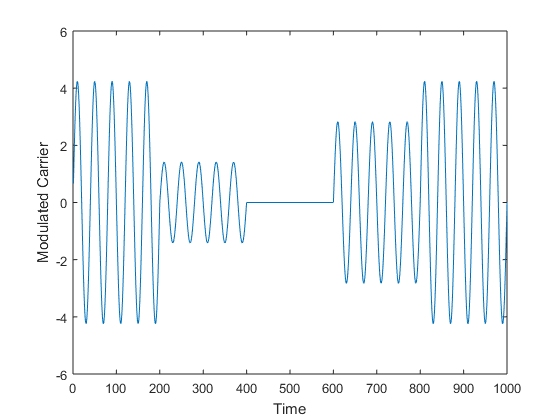••# Binary Amplitude Shift Keying

Amplitude Shift Keying (ASK) is a type of digital modulation in which the amplitude of the carrier is varied with respect to the digital message. If we send one bit/symbol, we call it as Binary Amplitude Shift Keying (BASK), in which case the carrier amplitude is assumed to have 2 values (one corresponding to 1 and other corresponding to 0). Figure below shows this type of modulation.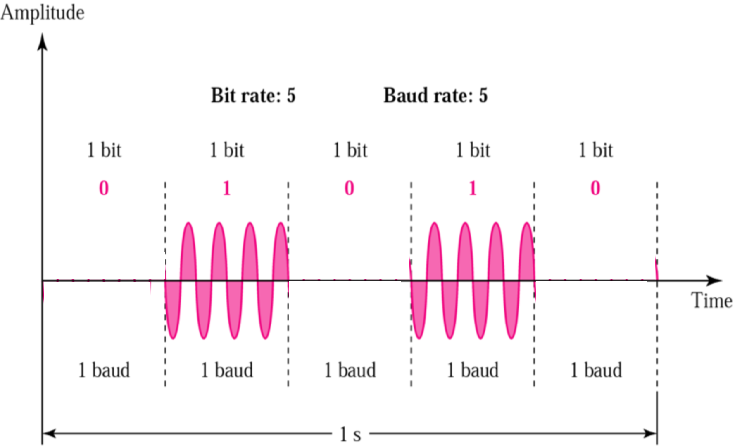This type of BASK is also called On-Off Keying (OOK) when one state is represented by absence of the carrier (0 amplitude), the corresponding constellation diagram is shown in Figure below, in which a signal is either present or absent.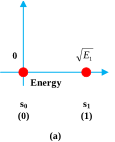A rather general case is shown in Figure below.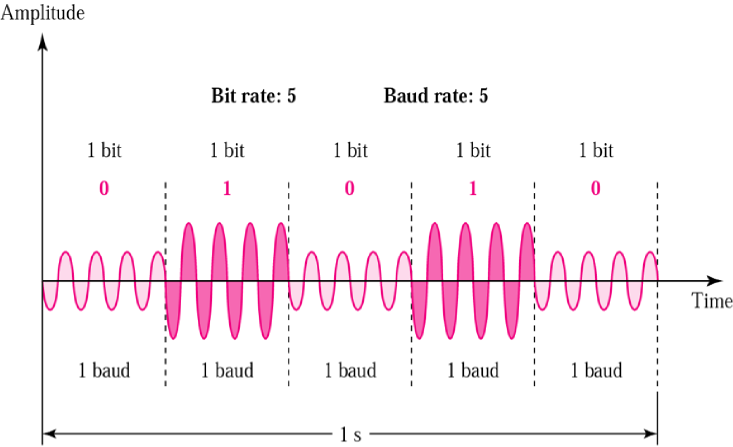And the constellation Diagram is shown as: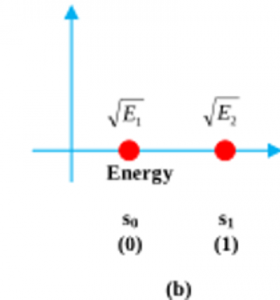Bit rate represents the No. of bits/sec whereas baud rate represents the No. of symbols sent per second. Baud rate is less than or equal to the bit rate. Modulated symbol waveform are represented as:$S_0=\sqrt{\frac{2E_0}{T}}\cos{(2\pi f_0 t)}\rightarrow represents \mbox{ }'0'$$S_1=\sqrt{\frac{2E_1}{T}}\cos{(2\pi f_0 t)}\rightarrow represents \mbox{ }'1'$

# Multiple Amplitude Shift Keying

Multiple Amplitude Shift Keying (MASK) refers to the modulation technique in which M different symbols are transmitted. Each of these symbols represents m bits of data where,$M=2^m$

and the corresponding value of m is calculated as,$m=log_2(M)$

A MASK modulated carrier may in general look like,$S_k(t)=\sqrt{\frac{2E_k}{T}}\cos{(2\pi f_0 t)} \mbox{ where } k=1,...,M$

# Basis Function Representation of MASK

Any MASK symbol can be assumed as a function and can be represented in terms of one basis function Ψ1(t). The basis function is given by,$\psi_1(t)=\sqrt{\frac{2}{T}}\cos{(2\pi f_0 t)}$$s_k(t)=\sqrt{E_k}\psi_1 (t)\mbox{ } k=1,...,M$

Different symbols are differentiated from each other based on the value of Ek, as the phase and frequency of the carrier remain same. Each symbol corresponds to a unique carrier amplitude. The resulting Constellation diagram of MASK signal is shown as: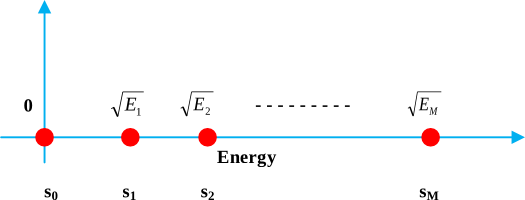Here is the example of 4-ASK signal

#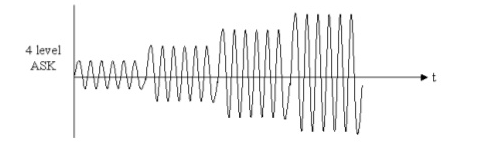A bit sequence is first converted to symbols, by a source encoder, based on the modulation scheme (value of M). For M=8, we would be sending three bits per symbol. There would be 8 symbols (waveforms) having 8 different amplitude levels corresponding to each 000, 001, 010, 011, 100, 101, 110, 111. Similarly for M=16; there will be 16 different waveforms corresponding to 0000 through 1111. This mapping of m bits to M voltage levels is achieved by the Pulse Amplitude Modulator Modulation (PAM) block.  This simulation of MASK uses M=4 i.e., we are using 4-ASK signal. Here, we are sending 2 bits per symbol and each of two bits can be mapped to any of 4 possible voltage levels. ’00’ bits is mapped to 0 volts, ’01’ bits is mapped to 1 volts, ’10’ bits is mapped to 2 volts, and ’11’ is mapped to 3 volts. Then the signal is modulated by multiplying it with basis function.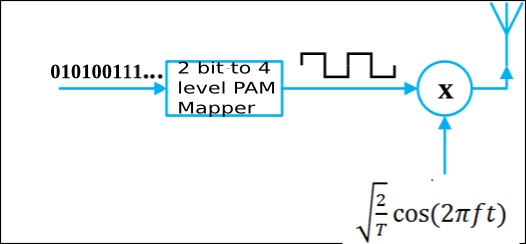We employ a coherent MASK demodulator. We assume that we have perfect phase information at the receiver for the sake of the simplicity. First the received signal is multiplied with the carrier (orthonormal basis function). Then the signal is integrated over T symbol duration T and sampled. After that the euclidean distance of this sample with all the possible PAM voltage levels (4 voltage levels in case of 4-ASK, as on the transmitter side) is calculated. And we choose the PAM voltage level having minimum euclidean distance. Then reverse mapping of that PAM voltage levels to bits is done.

# Matlab Code

The matlab code for modulation and demodulation of MASK (4ASK) is given below:

%_____________MASK Modulation and Demodulation Code________
clear all;
close all;
Nb=5;
Rb=1; %bps
T=1/Rb;

% Generate Nb bits randomly
%b =rand(1,Nb)&amp;amp;amp;gt;0.5;
b =[1 1 0 1 0 0 1 0 1 1];
%Rb is the bit rate in bits/second
bit_to_symbol=[];
for i=1:2:size(b,2)
a = [b(i) b(i+1)]
x = binaryVectorToDecimal(a)
switch(x)
case 
bit_to_symbol=[bit_to_symbol 0];
case 
bit_to_symbol=[bit_to_symbol 1];
case 
bit_to_symbol=[bit_to_symbol 2];
case 
bit_to_symbol=[bit_to_symbol 3];
end

end
NRZ_out=[];
RZ_out=[];
Manchester_out=[];
Vp=1;
for index=1:size(bit_to_symbol,2)
NRZ_out=[NRZ_out ones(1,200)*bit_to_symbol(index)];
end
figure;
stem(b, 'filled');
xlabel('Sequence Number')
ylabel ('Transmitted Bits [0/1]')
ylim ([0 2])
figure;
plot(NRZ_out, 'b', 'Linewidth', 2);
xlabel ('Time')
ylabel ('NRZ output')
ylim ([0 4])
grid on

t=0.005:0.005:5;
f=5;
Modulated=NRZ_out.*(sqrt(2/T)*sin(2*pi*f*t));
figure;
plot(Modulated);
xlabel ('Time')
ylabel ('Modulated Carrier')
ylim ([-6 6])

y=[];
demodulated=Modulated.*(sqrt(2/T)*sin(2*pi*f*t));
for i=1:200:size(demodulated,2)
y=[y trapz(t(i:i+199),demodulated(i:i+199))];
end

for (i=1:1:size(y,2))
euclidean_dist=sqrt((y(i)-[0 1 2 3]).^2);
[val index]=min(euclidean_dist)
temp = [0 1 2 3];
index = temp (index);
switch(index)
case 0
case 1
case 2
case 3
end

end

figure;
xlabel('Sequence Number')
ylim ([0 2])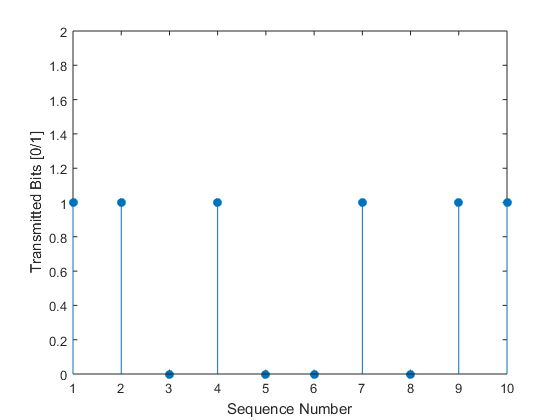Bits To Be transmitted

•Asim Zahid November 20, 2018, 7:43 pm